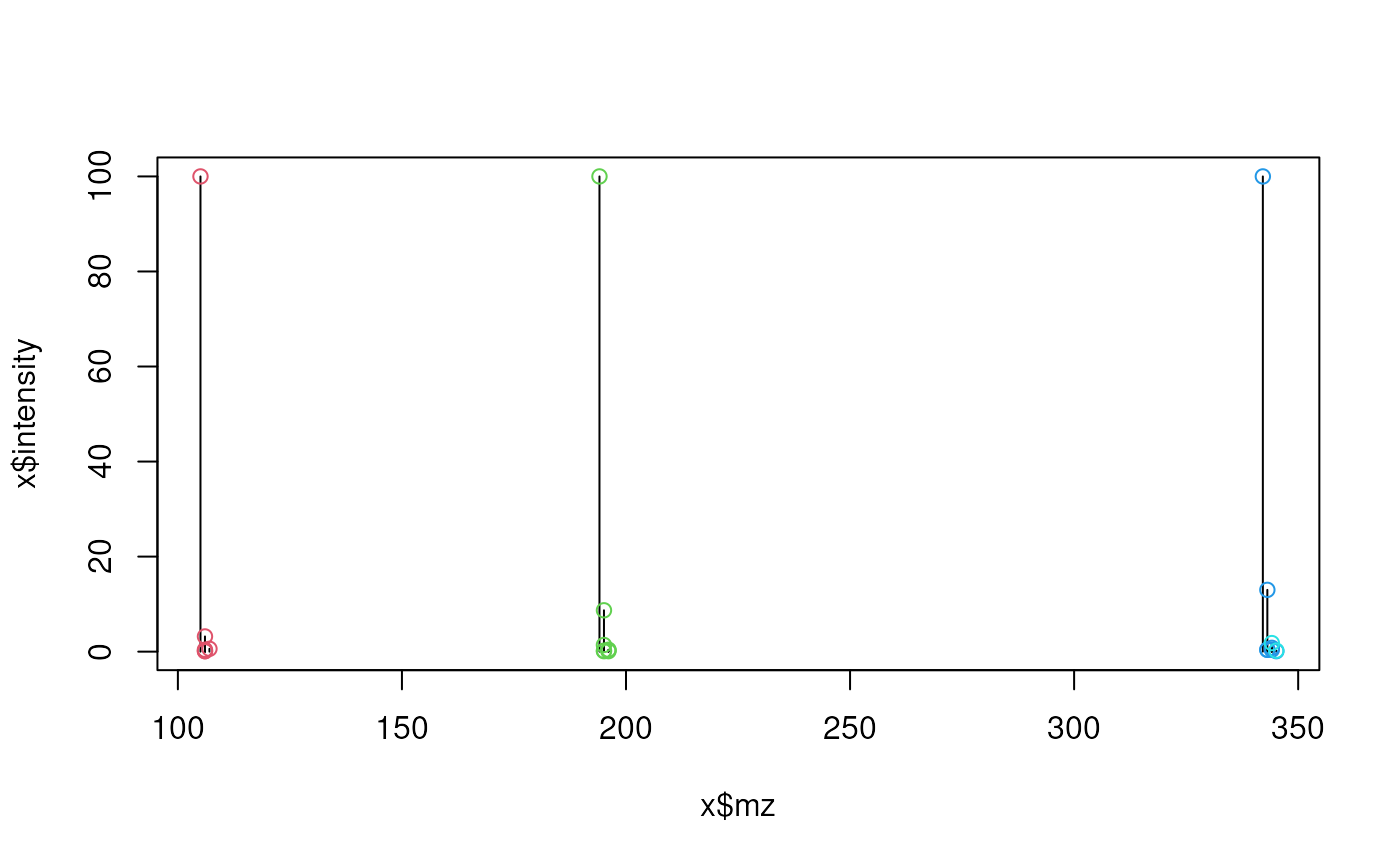Given a spectrum (i.e. a peak matrix with m/z and intensity values) the function identifies groups of potential isotopologue peaks based on pre-defined mass differences and intensity (probability) ratios that need to be passed to the function with the substDefinition parameter. Each isotopic substitution in a compound determines a certain isotopologue and it is associated with a certain mass difference of that with respect to the monoisotopic isotopologue. Also each substitution in a compound is linked to a certain ratio between the intensities of the peaks of the corresponding isotopologue and the monoisotopic one. This ratio isn't the same for isotopologues corresponding to the same isotopic substitution but to different compounds. Through the substDefinition parameter we provide upper and lower values to compute bounds for each isotopic substitution dependent on the peak's mass.

## Usage

isotopologues(
x,
substDefinition = isotopicSubstitutionMatrix(),
tolerance = 0,
ppm = 20,
seedMz = numeric(),
charge = 1
)

## Arguments

x

matrix with spectrum data (columns mz and intensity).

substDefinition

matrix or data.frame with definition of isotopic substitutions (columns "name" and "md" are among the mandatory columns). The rows in this matrix have to be ordered by column md in increasing order. See isotopicSubstitutionMatrix() for more information on the format and content.

tolerance

numeric(1) representing the absolute tolerance for the relaxed matching of m/z values of peaks. See MsCoreUtils::closest() for details.

ppm

numeric(1) representing a relative, value-specific parts-per-million (PPM) tolerance for the relaxed matching of m/z values of peaks. See MsCoreUtils::closest() for details.

seedMz

numeric vector of ordered m/z values. If provided, the function checks if there are peaks in x which m/z match them. If any, it looks for groups where the first peak is one of the matched ones.

charge

numeric(1) representing the expected charge of the ionized compounds.

## Value

list of integer vectors. Each integer vector contains the indixes of the rows in x with potential isotopologues of the same compound.

## Details

The function iterates over the peaks (rows) in x. For each peak (which is assumed to be the monoisotopic peak) it searches other peaks in x with a difference in mass matching (given ppm and tolerance) any of the pre-defined mass differences in substDefinitions (column "md"). The mass is obtained by multiplying the m/z of the peaks for the charge expected for the ionized compounds.

For matching peaks, the function next evaluates whether their intensity is within the expected (pre-defined) intensity range. Using "LBint", "LBslope", "UBint", "UBslope" of the previously matched isotopic substitution in substDefinition, the function estimates a (mass dependent) lower and upper intensity ratio limit based on the peak's mass.

When some peaks are grouped together their indexes are excluded from the set of indexes that are searched for further groups (i.e. peaks already assigned to an isotopologue group are not considered/tested again thus each peak can only be part of one isotopologue group).

Andrea Vicini

## Examples


## Read theoretical isotope pattern (high resolution) from example file
x <- x[order(x$mz), ] plot(x$mz, x$intensity, type = "h") isos <- isotopologues(x, ppm = 5) isos #> [] #>  1 2 3 4 5 6 #> #> [] #>  7 8 9 10 11 12 13 14 #> #> [] #>  15 16 17 18 20 21 24 #> #> [] #>  19 23 #> ## highlight them in the plot for (i in seq_along(isos)) { z <- isos[[i]] points(x$mz[z], x\$intensity[z], col = i + 1)